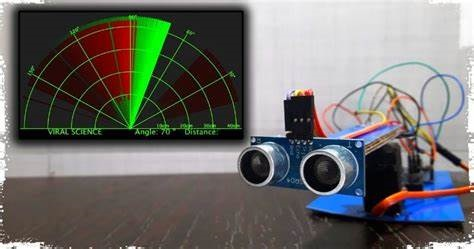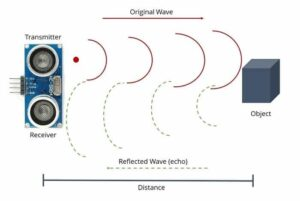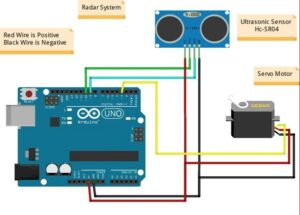• Post author:

RADAR project uses ultrasonic sensor to detect any object in its range and servo is used to rotate the ultrasonic sensor in 180 degree repeatedly to detect any object in 180 degree range.  It is just like mini radar it recognizes the object in the path and makes a red affected area near the object. so, this is called radar and it works on the Ultrasonic frequency. it consists ultrasonic sensor mounted over the servo motor and connected to software that shows the output on the computer screen. the interface of this software is pretty the same as the Radar interface.

### Components

Ardino Uno, Cable, Ultrasonic sensor, Servo motor, Lcd Display, wires and Processing Software

### How Does Radar using Arduino Works?

The ultrasonic sensor rotates with the servo motor and transmitted the ultrasonic waves (40 kHz) during this time. and the whole time a graph interface make in the simulation software. and if any object comes under the range of the ultrasonic sensor it starts to detect the object. at that time the graph interface inside the software becomes red in the object area. ultrasonic sensor work as an object detector in this project. Radar using ultrasonic sensor works in software makes the reaction according to the waves received.Ultrasonic sensors have two terminals one is a transmitter and another is the receiver. The transmitter terminal is known as the Trigger and the receiver terminal is known as the echo. Arduino continuously gives a command to the Servo motor to rotate. and the transmitter transmits the signal parallelly likewise the software also makes the graph. The ultrasonic sensor gave a different signal to the Arduino if anything comes in the path. then Arduino notifies the software for the affected region. the project depends on the ultrasonic sensor working. Radar using Arduino, ultrasonic sensor, and servo motor contents no other major components.### Distance Calculation

Duration = time-span between emitting the signal and receiving the echo.

The ultrasonic wave is basically a sound wave that travels at a speed of 340 m/s (0.034 cm/s). The ultrasonic sensor is measuring the time it takes to hit the object and then come back but we need only time that it takes to hit the object. So, we will divide it by 2.

Distance= duration*0.034/2;

### Ardino code

```// Includes the Servo vlibrary
#include <Servo.h>
#include <LiquidCrystal.h>
// Defines Tirg and Echo pins of the Ultrasonic Sensor
const int trigPin = 4;
const int echoPin = 6;
int led = 2;
LiquidCrystal lcd(A5,A4,A3,A2,A1,A0);
// Variables for the duration and the distance
long duration;
int distance;
// Creates a servo object for controlling the servo motor
Servo myServo;

void setup() {
lcd.begin(16,2);
pinMode(led, OUTPUT);
pinMode(trigPin, OUTPUT); // Sets the trigPin as an Output
pinMode(echoPin, INPUT); // Sets the echoPin as an Input
Serial.begin(9600);
myServo.attach(8); // Defines on which pin is the servo motor attached
lcd.print(" Ultra sonic");
lcd.setCursor(0,1);
delay(2000);
}
void loop() {
// rotates the servo motor from 15 to 165 degrees
for(int i=15;i<=165;i++){
myServo.write(i);
delay(30);
distance = calculateDistance();// Calls a function for calculating the distance measured by the Ultrasonic sensor for each degree

Serial.print(i); // Sends the current degree into the Serial Port
Serial.print(","); // Sends addition character right next to the previous value needed later in the Processing IDE for indexing
Serial.print(distance); // Sends the distance value into the Serial Port
Serial.print("."); // Sends addition character right next to the previous value needed later in the Processing IDE for indexing
lcd.clear();
lcd.print("Distance:");
lcd.print(distance); //Print distance in lcd
lcd.print("cm");
if( distance <= 10){
lcd.setCursor(0,2);
lcd.print("***- Danger -***"); //Print danger when range is below 10cm
digitalWrite(led, HIGH); // turn the LED on (HIGH is the voltage level)
}else{
digitalWrite(led, LOW); // turn the LED off by making the voltage LOW
}
}

// Repeats the previous lines from 165 to 15 degrees
for(int i=165;i>15;i--){
myServo.write(i);
delay(30);
distance = calculateDistance();
Serial.print(i);
Serial.print(",");
Serial.print(distance);
Serial.print(".");
lcd.clear();
lcd.print("Distance:");
lcd.print(distance);
lcd.print("cm");
if( distance <= 10){
lcd.setCursor(0,2);
lcd.print("***- Danger -***");
digitalWrite(led, HIGH); // turn the LED on (HIGH is the voltage level)
}else{
digitalWrite(led, LOW); // turn the LED off by making the voltage LOW
}

}
}
// Function for calculating the distance measured by the Ultrasonic sensor
int calculateDistance(){

digitalWrite(trigPin, LOW);
delayMicroseconds(2);
// Sets the trigPin on HIGH state for 10 micro seconds
digitalWrite(trigPin, HIGH);
delayMicroseconds(10);
digitalWrite(trigPin, LOW);
duration = pulseIn(echoPin, HIGH); // Reads the echoPin, returns the sound wave travel time in microseconds
distance= duration*0.034/2;
return distance;
}```

### Processing code

```import processing.serial.*; // imports library for serial communication
import java.awt.event.KeyEvent; // imports library for reading the data from the serial port
import java.io.IOException;
Serial myPort; // defines Object Serial
// defubes variables
String angle = "";
String distance = "";
String data = "";
String noObject;
float pixsDistance;
int iAngle, iDistance;
int index1 = 0;
int index2 = 0;
PFont orcFont;
void setup() {

size (1200, 700); // ***CHANGE THIS TO YOUR SCREEN RESOLUTION***
smooth();
myPort = new Serial(this, "COM6", 9600); // starts the serial communication /importANT
myPort.bufferUntil('.'); // reads the data from the serial port up to the character '.'. So actually it reads this: angle,distance.
}
void draw() {

fill(98, 245, 31);
// simulating motion blur and slow fade of the moving line
noStroke();
fill(0, 4);
rect(0, 0, width, height - height * 0.065);

fill(98, 245, 31); // green color
// calls the functions for drawing the radar
drawLine();
drawObject();
drawText();
}
void serialEvent (Serial myPort) { // starts reading data from the Serial Port
// reads the data from the Serial Port up to the character '.' and puts it into the String variable "data".
data = data.substring(0, data.length() - 1);

index1 = data.indexOf(","); // find the character ',' and puts it into the variable "index1"
angle = data.substring(0, index1); // read the data from position "0" to position of the variable index1 or thats the value of the angle the Arduino Board sent into the Serial Port
distance = data.substring(index1 + 1, data.length()); // read the data from position "index1" to the end of the data pr thats the value of the distance

// converts the String variables into Integer
iAngle = int(angle);
iDistance = int(distance);
}
pushMatrix();
translate(width / 2, height - height * 0.074); // moves the starting coordinats to new location
noFill();
strokeWeight(2);
stroke(98, 245, 31);
// draws the arc lines
arc(0, 0, (width - width * 0.0625), (width - width * 0.0625), PI, TWO_PI);
arc(0, 0, (width - width * 0.27), (width - width * 0.27), PI, TWO_PI);
arc(0, 0, (width - width * 0.479), (width - width * 0.479), PI, TWO_PI);
arc(0, 0, (width - width * 0.687), (width - width * 0.687), PI, TWO_PI);
// draws the angle lines
line(-width / 2, 0, width / 2, 0);
line((-width / 2)*cos(radians(30)), 0, width / 2, 0);
popMatrix();
}
void drawObject() {
pushMatrix();
translate(width / 2, height - height * 0.074); // moves the starting coordinats to new location
strokeWeight(9);
stroke(255, 10, 10); // red color
pixsDistance = iDistance * ((height - height * 0.1666) * 0.025); // covers the distance from the sensor from cm to pixels
// limiting the range to 40 cms
if (iDistance < 40) {
// draws the object according to the angle and the distance
}
popMatrix();
}
void drawLine() {
pushMatrix();
strokeWeight(9);
stroke(30, 250, 60);
translate(width / 2, height - height * 0.074); // moves the starting coordinats to new location
line(0, 0, (height - height * 0.12)*cos(radians(iAngle)), -(height - height * 0.12)*sin(radians(iAngle))); // draws the line according to the angle
popMatrix();
}
void drawText() { // draws the texts on the screen

pushMatrix();
if (iDistance > 40) {
noObject = "Out of Range";
}
else {
noObject = "In Range";
}
fill(0, 0, 0);
noStroke();
rect(0, height - height * 0.0648, width, height);
fill(98, 245, 31);
textSize(25);

text("10cm", width - width * 0.3854, height - height * 0.0833);
text("20cm", width - width * 0.281, height - height * 0.0833);
text("30cm", width - width * 0.177, height - height * 0.0833);
text("40cm", width - width * 0.0729, height - height * 0.0833);
textSize(40);
text("Pak Cyber Boy ", width - width * 0.875, height - height * 0.0277);
text("Angle: " + iAngle + " °", width - width * 0.48, height - height * 0.0277);
text("Distance: ", width - width * 0.26, height - height * 0.0277);
if (iDistance < 40) {
text(" " + iDistance + " cm", width - width * 0.225, height - height * 0.0277);
}
textSize(25);
fill(98, 245, 60);
translate((width - width * 0.4994) + width / 2 * cos(radians(30)), (height - height * 0.0907) - width / 2 * sin(radians(30)));
text("30°", 0, 0);
resetMatrix();
translate((width - width * 0.503) + width / 2 * cos(radians(60)), (height - height * 0.0888) - width / 2 * sin(radians(60)));
text("60°", 0, 0);
resetMatrix();
translate((width - width * 0.507) + width / 2 * cos(radians(90)), (height - height * 0.0833) - width / 2 * sin(radians(90)));